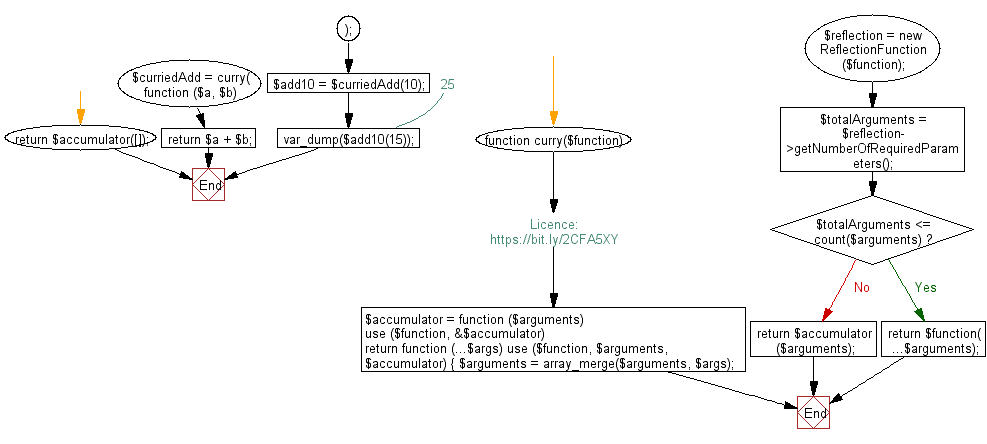﻿ PHP Exercise: Curry a function to take arguments in multiple calls - w3resource

# PHP Exercises: Curry a function to take arguments in multiple calls

## PHP: Exercise-100 with Solution

Write a PHP program to curry a function to take arguments in multiple calls.

Sample Solution: -

PHP Code:

``````<?php
//Licence: https://bit.ly/2CFA5XY

function curry(\$function)
{
\$accumulator = function (\$arguments) use (\$function, &\$accumulator) {
return function (...\$args) use (\$function, \$arguments, \$accumulator) {
\$arguments = array_merge(\$arguments, \$args);
\$reflection = new ReflectionFunction(\$function);
\$totalArguments = \$reflection->getNumberOfRequiredParameters();

if (\$totalArguments <= count(\$arguments)) {
return \$function(...\$arguments);
}

return \$accumulator(\$arguments);
};
};

return \$accumulator([]);
}

function (\$a, \$b) {
return \$a + \$b;
}
);

?>
```
```

Sample Output:

```int(25)
```

Flowchart:PHP Code Editor:

Have another way to solve this solution? Contribute your code (and comments) through Disqus.

What is the difficulty level of this exercise?

Test your Programming skills with w3resource's quiz.

﻿

## PHP: Tips of the Day

Returns all elements in an array except for the first one

Example:

```<?php
function tips_tail(\$items)
{
return count(\$items) > 1 ? array_slice(\$items, 1) : \$items;
}

print_r(tips_tail([1, 5, 7]));
?>
```

Output:

```Array
(
 => 5
 => 7
)
```